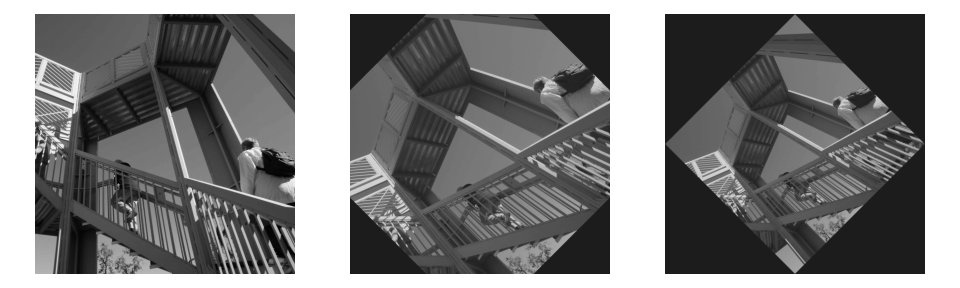# scipy.ndimage.rotate¶

scipy.ndimage.rotate(input, angle, axes=1, 0, reshape=True, output=None, order=3, mode='constant', cval=0.0, prefilter=True)[source]

Rotate an array.

The array is rotated in the plane defined by the two axes given by the axes parameter using spline interpolation of the requested order.

Parameters
inputarray_like

The input array.

anglefloat

The rotation angle in degrees.

axestuple of 2 ints, optional

The two axes that define the plane of rotation. Default is the first two axes.

reshapebool, optional

If reshape is true, the output shape is adapted so that the input array is contained completely in the output. Default is True.

outputarray or dtype, optional

The array in which to place the output, or the dtype of the returned array. By default an array of the same dtype as input will be created.

orderint, optional

The order of the spline interpolation, default is 3. The order has to be in the range 0-5.

mode{‘reflect’, ‘constant’, ‘nearest’, ‘mirror’, ‘wrap’}, optional

The mode parameter determines how the input array is extended beyond its boundaries. Default is ‘constant’. Behavior for each valid value is as follows:

‘reflect’ (d c b a | a b c d | d c b a)

The input is extended by reflecting about the edge of the last pixel.

‘constant’ (k k k k | a b c d | k k k k)

The input is extended by filling all values beyond the edge with the same constant value, defined by the cval parameter.

‘nearest’ (a a a a | a b c d | d d d d)

The input is extended by replicating the last pixel.

‘mirror’ (d c b | a b c d | c b a)

The input is extended by reflecting about the center of the last pixel.

‘wrap’ (a b c d | a b c d | a b c d)

The input is extended by wrapping around to the opposite edge.

cvalscalar, optional

Value to fill past edges of input if mode is ‘constant’. Default is 0.0.

prefilterbool, optional

Determines if the input array is prefiltered with spline_filter before interpolation. The default is True, which will create a temporary float64 array of filtered values if order > 1. If setting this to False, the output will be slightly blurred if order > 1, unless the input is prefiltered, i.e. it is the result of calling spline_filter on the original input.

Returns
rotatendarray

The rotated input.

Examples

>>> from scipy import ndimage, misc
>>> import matplotlib.pyplot as plt
>>> fig = plt.figure(figsize=(10, 3))
>>> ax1, ax2, ax3 = fig.subplots(1, 3)
>>> img = misc.ascent()
>>> img_45 = ndimage.rotate(img, 45, reshape=False)
>>> full_img_45 = ndimage.rotate(img, 45, reshape=True)
>>> ax1.imshow(img, cmap='gray')
>>> ax1.set_axis_off()
>>> ax2.imshow(img_45, cmap='gray')
>>> ax2.set_axis_off()
>>> ax3.imshow(full_img_45, cmap='gray')
>>> ax3.set_axis_off()
>>> fig.set_tight_layout(True)
>>> plt.show()>>> print(img.shape)
(512, 512)
>>> print(img_45.shape)
(512, 512)
>>> print(full_img_45.shape)
(724, 724)


#### Previous topic

scipy.ndimage.map_coordinates

#### Next topic

scipy.ndimage.shift